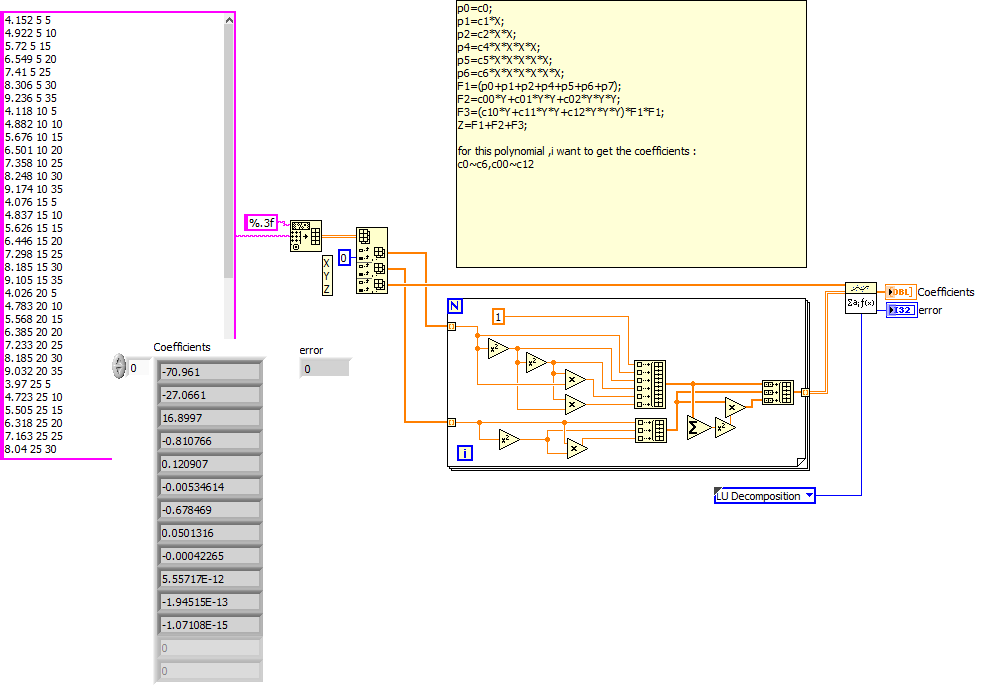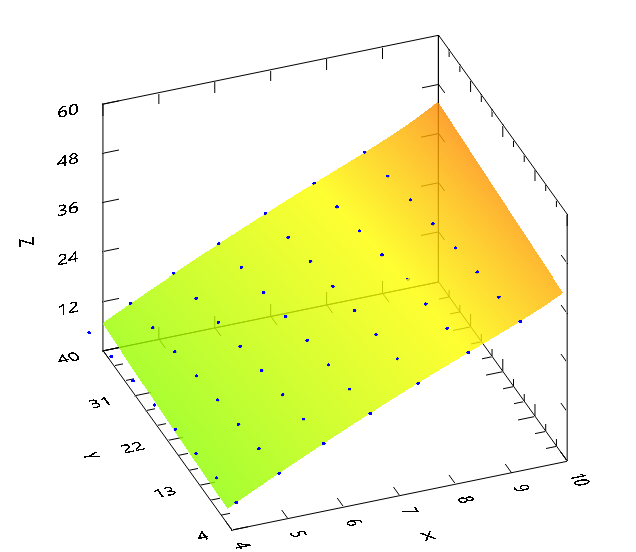# LabVIEW

cancel
Showing results for
Did you mean:

Solved!
Go to solution

## User defined high degree polynomial fit (surface fitting)

when we get data from the measurement ,we always meet the situation that the result value Z is related to 2 Independent Variable ,actually it likes 3D surface .

the formula always like :Z=F(X,Y).

I checked the  polynomial fit express vi on labview , but i still can not find a solution to built an complicated user-defined polynomial and get the coefficient.

for example ,i can defined a simple polynomial and let the X,Y,Z to fit as this polynomial ,then get coefficients.

this picture shows my defined polynomial Z=c0+c1*X+C2*X^2+C3*X^3+C4*X^4+C5*X^5+C00*Y+C01*Y^2+C02*Y^3

but the coefficient come form the every different degree formula ,then if i want to define a polynomial like :

p0=c0;
p1=c1*X;
p2=c2*X*X;
p4=c4*X*X*X*X;
p5=c5*X*X*X*X*X;
p6=c6*X*X*X*X*X*X;
F1=(p0+p1+p2+p4+p5+p6+p7);
F2=c00*Y+c01*Y*Y+c02*Y*Y*Y;
F3=(c10*Y+c11*Y*Y+c12*Y*Y*Y)*F1*F1;

Z=F1+F2+F3;

HOW CAN I TO  DEFINE this polynomial and fit as least square method ?

Thanks ,Message 1 of 15
(4,246 Views)

## 回复： User defined high degree polynomial fit (surface fitting)

i really need to get the coefficient after fitting !

Message 2 of 15
(4,245 Views)

## 回复： User defined high degree polynomial fit (surface fitting)

Can you attach your actual VI?LabVIEW Champion.Message 3 of 15
(4,217 Views)

## 回复： User defined high degree polynomial fit (surface fitting)

thank you for your reply , actually i learned this method from here ,named:"9P interpolacija v2.vi" ,i also attached it ,i downloaded it from here .

it's very normal to calculate the value by least square method in industry  ,for example :when you measure  the concentration of liquid via the  speed of sound  principle ,the concentration are  related to the speed of sound and temperature .so,the Z  is concentration and X is speed of sound ,Y is temperature .

sometimes ,you need to find a best model of surface to describe this relation .so you need to test different model of polynomial .

that is the reason why i need the coefficient .

thanks again

Message 4 of 15
(4,200 Views)

## 回复： User defined high degree polynomial fit (surface fitting)

Your VI does not contain any data. Please attach some reasonable xyz data to be fit.

(I cannot open your VI on english Windows unless I rename the file. Please use regular file names).

There are probably better ways to create the H Matrix than with a formula node. That 9P example looks overly complicated. But yes, you'll get the least square fit and coefficients out of it at the end.LabVIEW Champion.Message 5 of 15
(4,178 Views)

## 回复： User defined high degree polynomial fit (surface fitting)

here i make a modification on my vi ,thank you for your help kindly .

Message 6 of 15
(4,159 Views)
Solution
Accepted by topic author fyang2017

## 回复： User defined high degree polynomial fit (surface fitting)

Maybe something like this?

(Please double-check the math for the H matrix.)LabVIEW Champion.Message 7 of 15
(4,140 Views)

## 回复： User defined high degree polynomial fit (surface fitting)

Thank you  very very much ,this is really good solution for this kind of formula .i start to understanding the matrix in labview  via this example ,thanks  again.

i try to draw  a 3D surface by this data ,but the surface looks very strange !

Message 8 of 15
(4,115 Views)

## 回复： User defined high degree polynomial fit (surface fitting)

The H matrix won't give you any interesting surface. What you need to do is generate a xy grid that covers a similar range (in x and y) as the input data and is spaced sufficiently to show all the details, then calculate Z for each grid point. Overlay it over the real data.LabVIEW Champion.Message 9 of 15
(4,108 Views)

## 回复： User defined high degree polynomial fit (surface fitting)

I quickly modified one of my ancient VIs to show the general procedure. See if this works for you.

(This is using the old actvieX 3D graphs. Should be easy to adapt to the new versions)

(Note that SVD has problems. You need to use a different algorithm, such as LU decomposition)LabVIEW Champion.Message 10 of 15
(4,100 Views)